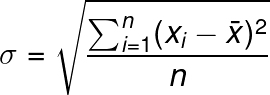# Mean Absolute Deviation Calculator

Or input your data as csv

Sharing helps us build more free tools

Mean absolute deviation is a measure of spread similar to the standard deviation or variance

Use this calculator to find the mean absolute deviation of an input data set

## Mean Absolute Deviation Formula

The mean absolute deviation is calculated as the average distance between each point x and the mean of x:## What is the Difference Between Mean Absolute Deviation and Standard Deviation

Mean absolute deviation and standard deviation are both measures of spread. They are both calculated based on the distance between a point and the mean of the points.

The standard deviation is calculated using the distance between the point and the mean of all points squared. While the mean absolute deviation is calculated using the distance between each point and the mean of all points.

The standard deviation formula is as follows:Notice that the standard deviation formula squares the distance between each point x and the mean of all points x. This means that outlier points x can greatly impact the standard deviation.

The mean absolute deviation does not use the square difference between each point and the mean of all points. It just uses the absolute distance. This means that the mean absolute deviation is less sensitive to outliers than the standard deviation.